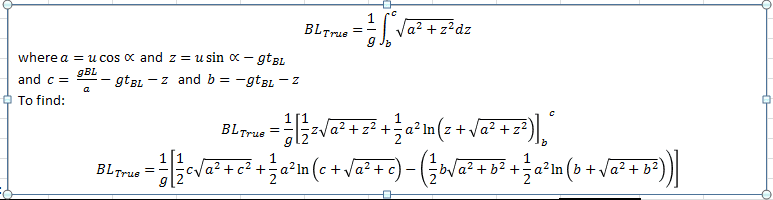# Finding Arc Length when given velocity and launch angle

## Homework Statement

Find the arc length of the projectile from launch until the time it hits the ground, given that
0 V is 100 feet/sec and is 45 degrees.

## Homework Equations

Arc Length= ∫_a^b▒√(█(1+(f^' (x) )^2@)) dx
Arc Length of Curve= ∫_a^b▒〖v(t)dt=∫_a^b▒√((dx/dt)^2+(dy/dt) )〗^2 dt

i know these aren't pretty versions of the arc length equations, sorry about that.

## The Attempt at a Solution

i'm not really sure how to go about plugging in what i was given into the equation. need help ASAP. thank you

Last edited:

Dick
Homework Helper
I'd suggest using the sqrt(x'(t)^2+y'(t)^2)*dt form. What are x'(t) and y'(t) and what are the limits on t? You'll get an integral you can use a trig (or hyperbolic trig) substitution on. Just to warn you, this isn't a terribly easy problem.

Last edited:
Hey,

This reply might be a bit late but could help others who are looking for information on this kind of problem. I am a chemical engineering student working on a project for which I am designing a laminar nozzle. I needed to find the break up length (arc length to which the jet begins to break up - laminar to turbulent) of the jet of water fired from the nozzle from the angle of the initial jet, the velocity of the jet and the horizontal length of the break up point from the nozzle outlet. I have devised the following equation for finding this arc length from projectile motion physics:

X = jet break up point/point along arc we wish to find the arc length to from origin
BL = horizontal break up length/x axis displacement of point X
θ = jet angle
u = jet velocity

so initially tBL = BL/u gives the time at which the jet breaks up (time to arc point X)
From projectile motion arc length can be shown as:

L = ∫√(ux2 + uy2) dt from t=0 to t=tBL (or any time chosen)
L = ∫√((ucosθ)2 + (usinθ - gt)2) dt

To make things easier:
a = ucosθ
z = usinθ - gt
Giving:

L = 1/g ∫√(a2 + z2) dz
1/g since dt = 1/g*dz

converting the integral boundaries:
when t = 0; z = -usinθ
when t = tBL:
Sx = utcosθ → BL = utBLcosθ (x-axis displacement of point X)
tBL = BL/(ucosθ)
∴ z = gBL/(ucosθ) - usinθ

Simplifying the boundaries:
when t = 0; -usinθ = -gtBL - z = b
when t = tBL; g*BL/(ucosθ) - usinθ = gBL/a - gtBL - z = c

Finally once the arc length equation is integrated:
L = 1/g[0.5*z*√(a2+z2) + 0.5*a2* ln(z + √(a2+z2))]bc
(b & c being the integral boundaries)

Once b & c are substituted into the equation in place of "z", L will be the length of the arc to point X along the parabola.

Hope this helps!

Here's a screen shot from excel of the equation if the above text is hard to follow:(in there there should be c2 under the square root which I now noticed I missed)

Keep in mind this is to find the length to a point X, there are far easier ways to find the total parabolic arc length (from origin(0,0) until y = 0 again)